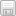# 2 multiple choice questions math homework help

i have 20 question multiple choice in precalculus

#### Question 1 (4 points)Use the slope and y-intercept to graph the linear function.G(x) = 3xQuestion 1 options:

 A)B)C)D)Save

#### Question 2 (4 points)Solve the inequality.x2 + 6x â‰¤ 0

Question 2 options:

 A) [-6, 0]

 B) (-âˆž, 0] or [6, âˆž)

 C) (-âˆž, -6] or [0, âˆž)

 D) [0, 6]

Save

#### Question 3 (4 points)Use the figure to solve the inequality.f(x) < 0Question 3 options:

 A) {x|x â‰¤ -5 or x â‰¥ 3}; (-âˆž, -5] or [3, âˆž)

 B) {x|-5 < x < 3}; (-5, 3)

 C) {x|-5 â‰¤ x â‰¤ 3}; [-5, 3]

 D) {x|x < -5 or x > 3}; (-âˆž, -5) or (3, âˆž)

Save

#### Question 4 (4 points)Use the slope and y-intercept to graph the linear function.p(x) = -x – 3Question 4 options:

 A)B)C)D)Save

#### Question 5 (4 points)Determine, without graphing, whether the given quadratic function has a maximum value or a minimum value and then find that value.f(x) = x2 – 8

Question 5 options:

 A) maximum; -8

 B) minimum; -8

 C) minimum; 0

 D) maximum; 0

Save

#### Question 6 (4 points)Determine the slope and y-intercept of the function.p(x) = -x + 4

Question 6 options:

 A) m = -1; b = – 4

 B) m = 0; b = 4

 C) m =1; b = – 4

 D) m = -1; b =4

Save

#### Question 7 (4 points)Use the slope and y-intercept to graph the linear function.F(x) = 6Question 7 options:

 A)B)C)D)Save

#### Question 8 (4 points)Solve the inequality.x2 – 2x – 15 â‰¤ 0

Question 8 options:

 A) (-âˆž, -3] or [5, âˆž)

 B) [-3, 5]

 C) [5, âˆž)

 D) (-âˆž, -3]

Save

#### Question 9 (4 points)Solve the problem.The manufacturer of a CD player has found that the revenue R (in dollars) iswhen the unit price is p dollars. If the manufacturer sets the price p to maximize revenue, what is the maximum revenue to the nearest whole dollar?

Question 9 options:

 A) \$194,045

 B) \$388,090

 C) \$1,552,360

 D) \$776,180

Save

#### Question 10 (4 points)Solve the inequality.x2 + 3x – 4 > 0

Question 10 options:

 A) (-âˆž, -4)

 B) (-4, 1)

 C) (1, âˆž)

 D) (-âˆž, -4) or (1, âˆž)

Save

#### Question 11 (4 points)Graph the function using its vertex, axis of symmetry, and intercepts.f(x) = -x2 + 4xQuestion 11 options:

 A) vertex (2, -4)intercept (0, -8)B) vertex (-2, 4)intercepts (0, 0), (- 4, 0)C) vertex (2, 4)intercepts (0, 0), (4, 0)D) vertex (-2, -4)intercept (0, -8)Save

#### Question 12 (4 points)Solve the problem.The owner of a video store has determined that the cost C, in dollars, of operating the store is approximately given bywhere x is the number of videos rented daily. Find the lowest cost to the nearest dollar.

Question 12 options:

 A) \$741

 B) \$559

 C) \$438

 D) \$620

Save

#### Question 13 (4 points)Determine, without graphing, whether the given quadratic function has a maximum value or a minimum value and then find that value.f(x) = -x2 – 2x + 1

Question 13 options:

 A) minimum; – 1

 B) minimum; 2

 C) maximum; 2

 D) maximum; – 1

Save

#### Question 14 (4 points)Determine the quadratic function whose graph is given.
||

Question 14 options:

 A) f(x) = -x2 – 4x – 5

 B) f(x) = -x2 + 4x + 5

 C) f(x) = x2 – 8x + 5

 D) f(x) = x2 + 4x + 5

Save

#### Question 15 (4 points)Determine the slope and y-intercept of the function.G(x) = -6x

Question 15 options:

 A) m = 0; b = -6

 B) m = -6; b = 0